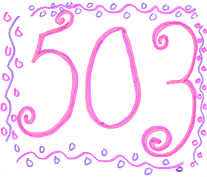# 503

This number is a prime.Heather Lundy © 2002The smallest prime that is the sum of cubes of the first n primes (23 + 33 + 53 + 73). [Honaker, 2000]According to the 2000 Wisden, the prime number 503 was the number of hours of sunshine in England's gloomiest summer of the 20th century (1954).503^5 + 503^0 + 503^3 is prime. It is the smallest prime with this property. [Firoozbakht]Clark Kent's Metropolis apartment number in the "Superman" series. [Morse]5! + 0! + 3! is a three-digit Mersenne prime. [Morse]Theatre503 is a performing arts venue in London that specializes in new work.With 509, the smallest pair of consecutive sexy Cyclops primes. [Punches]"503 Service Unavailable" is a HTTP error code that indicates a temporary server failure state due to overload or maintenance. [Beedassy]The smallest prime of the form 2x-x, where x is a composite number. [Loungrides]The only 3-digit Cyclops prime of form p0q, where p, q are consecutive primes, i.e., (3, 5). [Loungrides]Smallest (only?) prime of form 2^(p^2) - p^2, where p is prime. [Srivastava]Almost certainly the smallest prime p such that neither pk - 1 nor pk + 1 have exactly k prime factors counting multiplicity. [Merickel]D-503 is the name of the mathematician and main character of the novel "We" written by Yevgeny Zamyatian, which is a classic in Russian Literature. [Iglesias]The sum of the first ten positive integers that have sums of digits of eight is a prime number (503) with a sum of digits of eight. [Gaydos]

(There are 6 curios for this number that have not yet been approved by an editor.)